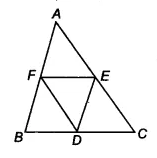Q

# D, E and F are respectively the mid-points of the sides BC, CA and AB of a ∆ ABC. Show that (iii) ar (BDEF) = 1/2 ar (ABC)

Q: 5    D, E and F are respectively the mid-points of the sides BC, CA and AB of a . Show that

(iii)

Viewsar(DEF) = ar ( BDF).........(i)

So, ar(||gm BDEF) = ar(BDF) + ar (DEF)
= 2 . ar(DEF)    [from equation (i)]

Hence proved.

Exams
Articles
Questions